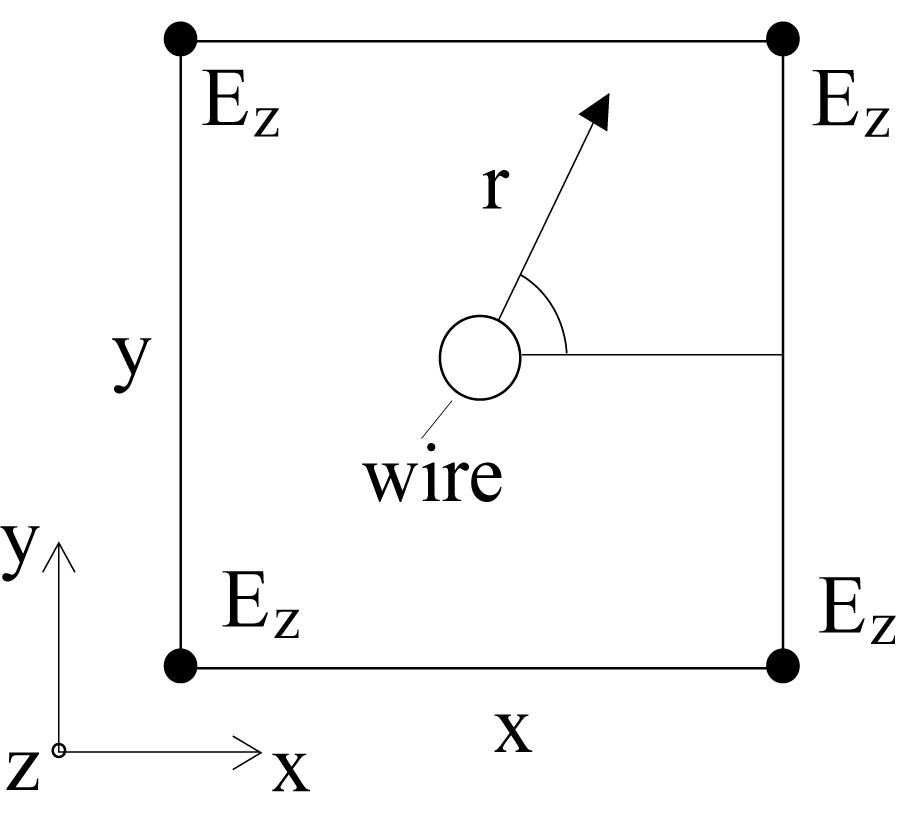Vol. 51
Latest Volume
All Volumes
All Journals
2016-11-06
PIER M
Vol. 51, 195-203, 2016
Diagnosis of Coupled Resonator Bandpass Filters Using VF and Optimization Method
Rui Wang , Le-Zhong Li , Long Peng , Xiao-Qiang Tu and Xiao-Xi Zhong
This paper presents a hybrid method combining a vector fitting (VF) and a global optimization for diagnosing coupled resonator bandpass filters. The method can extract coupling matrix from the measured or the electromagnetically simulated admittance parameters (Y-parameters) of a narrow band coupled resonator bandpass filter with losses. The optimization method is used to remove the phase shift effects of the measured or the EM simulated Y-parameters caused by the loaded transmission lines at the input/output ports of a filter. VF is applied to determine the complex poles and residues of the Y-parameters without phase shift. The coupling matrix can be extracted (also called the filter diagnosis) by these complex poles and residues. The method can be used to computer-aided tuning (CAT) of a filter in the stage of this filter design and/or product process to accelerate its physical design. Three application examples illustrate the validity of the proposed method.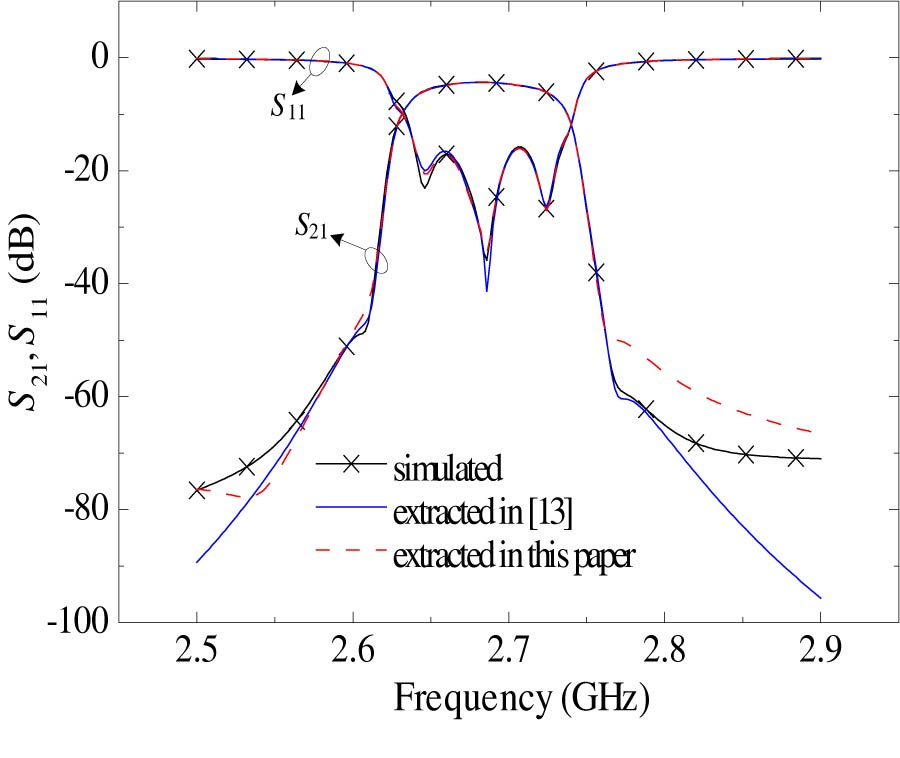2016-11-04
PIER M
Vol. 51, 185-194, 2016
A Novel Micro-g Emulation System Using Active Magnetic Compensator for Complex Space Operations
Tao Wen , Zhengfeng Ming , Zhanxia Zhu , Wenzhi Zhu and Shuang Ning
To perform the ground simulation experiments of the complex space operations, this work proposes a new active magnetic suspension compensator. The large-gap magnetic suspension compensator (LGMSC) is a conceptual design for a ground-based experiment which could be used to investigate the technology issues associated with accurate suspended element control at large gaps. This compensator can be used as the out-of-plane electromagnetic actuator for the 3-DOF fine stage in certain high precision positioning applications. Based on the equivalent current method, we explain the basics of the magnetic suspension compensator and analyze its advantages. A gravity compensator has been realized in a test setup that shows the feasibility of the chosen modeling technique and of magnetic gravity compensation.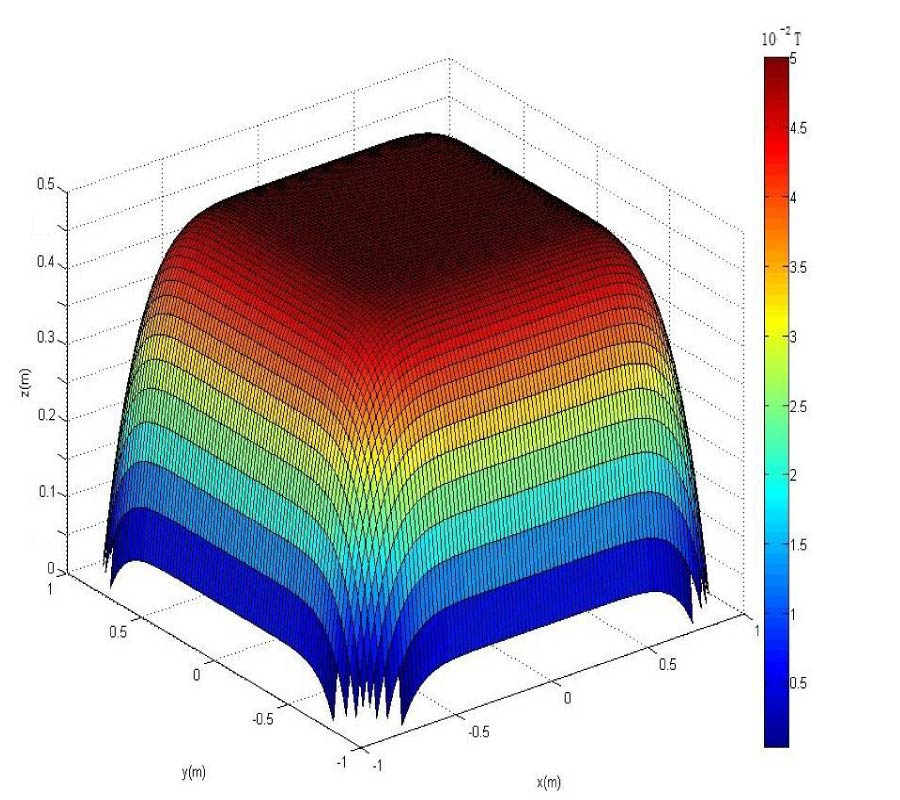2016-11-04
PIER M
Vol. 51, 175-183, 2016
Radiation Field and Optical Coupling Evaluation Using a New Mathematical Model
Mansour Bacha and Abderrahmane Belghoraf
The mathematical model elaborated in this paper is based on the concept of intrinsic modes in order to analyze and synthesize optical wave propagation along a non-uniform optical structure which is used in integrated optics communication as tapered optical coupler. The new mathematical model is simply developed by introducing modifications to the intrinsic integral, and its numerical evaluation illustrate the electromagnetic field distribution inside a taper thin film and also outside the waveguide constituted by the substrate and the cladding of lower refractive index. The proposed method permits efficiently tracking the behaviour of the optical waves both inside and outside of the optical waveguide, and quantifying the radiation and optical coupling occurring from the taper thin film of higher refractive index to adjacent mediums until a total energy transfer; this happens at thicknesses lower than waveguide cutoff thickness of each mode. The new model can be applied to all types of tapered optical coupler, having a high or low contrast of the refractive indexes, and different wedge angles formed by the different mediums of the waveguide.2016-11-04
PIER M
Vol. 51, 165-173, 2016
Application of Group Theory for Computation Reduction in Microwave Imaging of Human Breast Model at 500 MHz
Hardik N. Patel and Deepak Ghodgaonkar
In microwave imaging, accuracy of breast cancer detection depends on complex permittivity profile reconstruction in breast. Inverse scattering problem is solved to reconstruct complex permittivity profile of breast. In this paper, computation time to solve inverse scattering problem is reduced by exploiting symmetry present in breast models using group theory. Forward problem is solved using method of moments. Levenberg-Marquardt algorithm is used to solve inverse scattering problem with and without group theory. Results show that computation time is reduced considerably by exploiting symmetry present in breast models using group theory. At higher SNR, error in complex permittivity reconstruction with group theory is approximately same as error without group theory.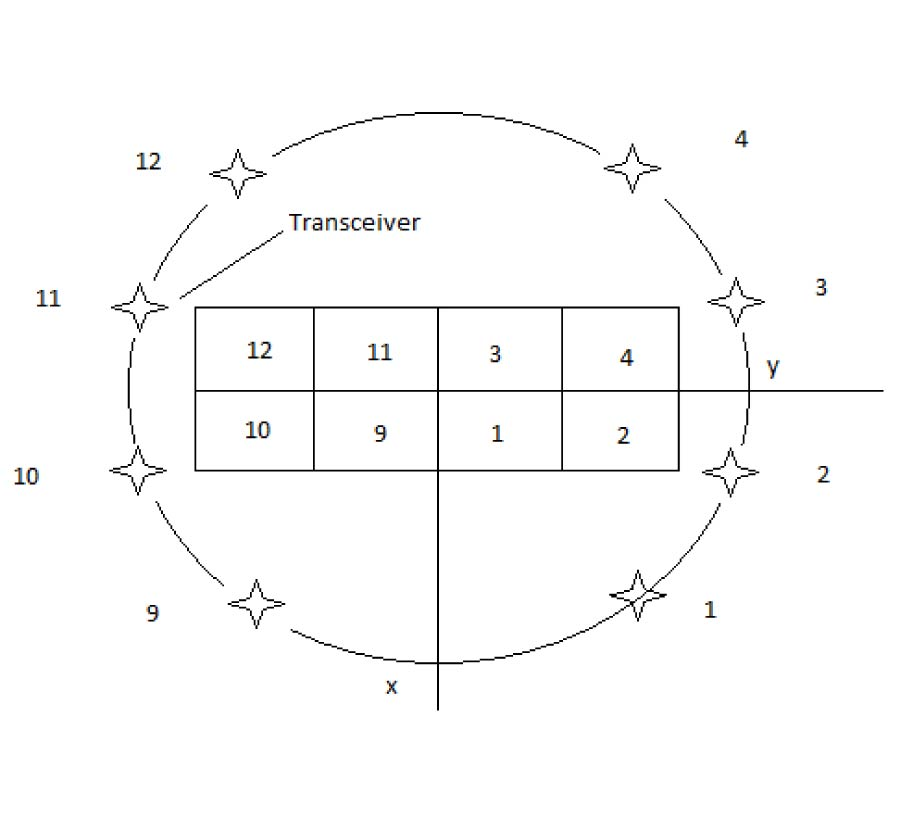2016-11-03
PIER M
Vol. 51, 157-163, 2016
Electromagnetic Waves Radiation by a Vibrators System with Variable Surface Impedance
Sergey L. Berdnik , Viktor A. Katrich , Mikhail Nesterenko and Yuriy M. Penkin
The problem of electromagnetic waves radiation by a vibrators system with variable distributed surface impedance along their axes located in free space is solved by the generalized method of induced electromotive forces (EMF). The distinctive peculiarity of this method is the use of the functional distributions, obtained as a result of the analytical solution of the integral equation for the current by the asymptotic averaging method before, as the basic approximations for the currents along the impedance vibrators. The multi-parameter characteristics of three-element and multi-element antennas with variable impedance vibrators are calculated.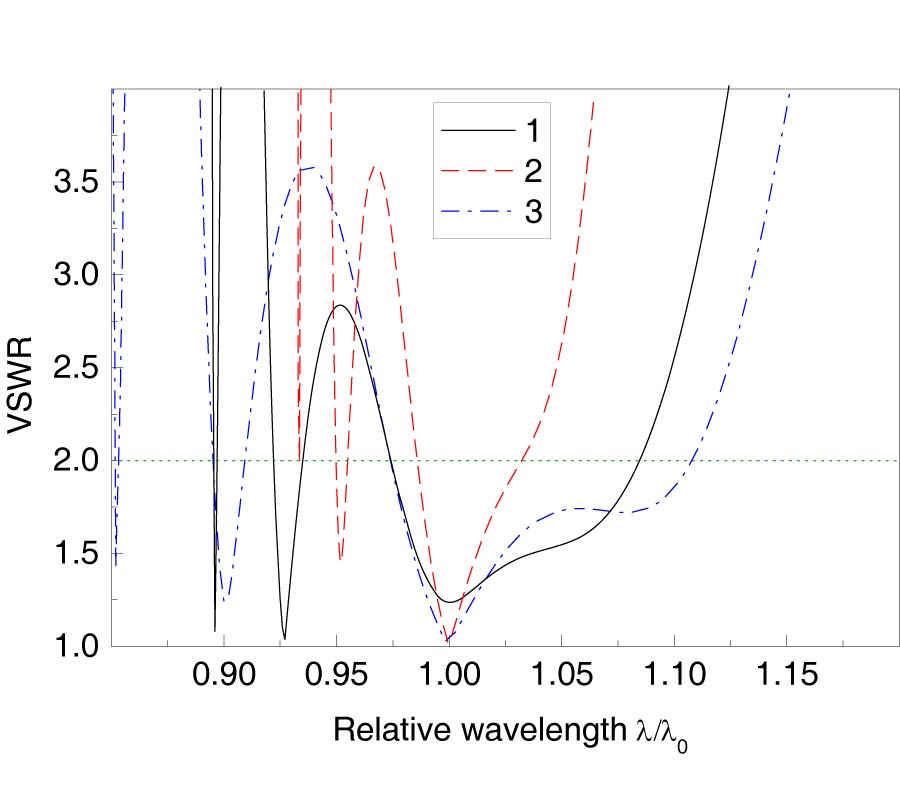2016-10-31
PIER M
Vol. 51, 147-156, 2016
Design and Analysis of Novel Bearingless Permanent Magnet Synchronous Motor for Flywheel Energy Storage System
Huangqiu Zhu and Ronghua Lu
To effectively simplify system structure and improve power density and efficiency, a design for a motor/generator suitable for flywheel energy storage system (FESS) is proposed. The machine is an outer-rotor and coreless-stator-type bearingless permanent magnet synchronous motor (BPMSM) with a Halbach array. Firstly, the operation principle of the outer-rotor BPMSM is described. Then, the structure and performance of the Halbach permanent magnet (PM) array are analyzed. The airgap magnetic field, back-EMF, suspension force, unilateral magnetic pull force and electromagnetic torque are calculated and analyzed by the finite element analysis (FEA). Finally, it is verified that the magnetic field of the suspension force windings increases the rotor eddy current loss by transient FEA coupled with external circuit. The rotor eddy current losses of BPMSM with different structures are compared. The comparison results show that the rotor eddy current loss of the coreless-stator-type BPMSM with Halbach array is the lowest. The simulation results verify the theoretical analysis and structure design, which can provide reference for the application of the motor in the FESS.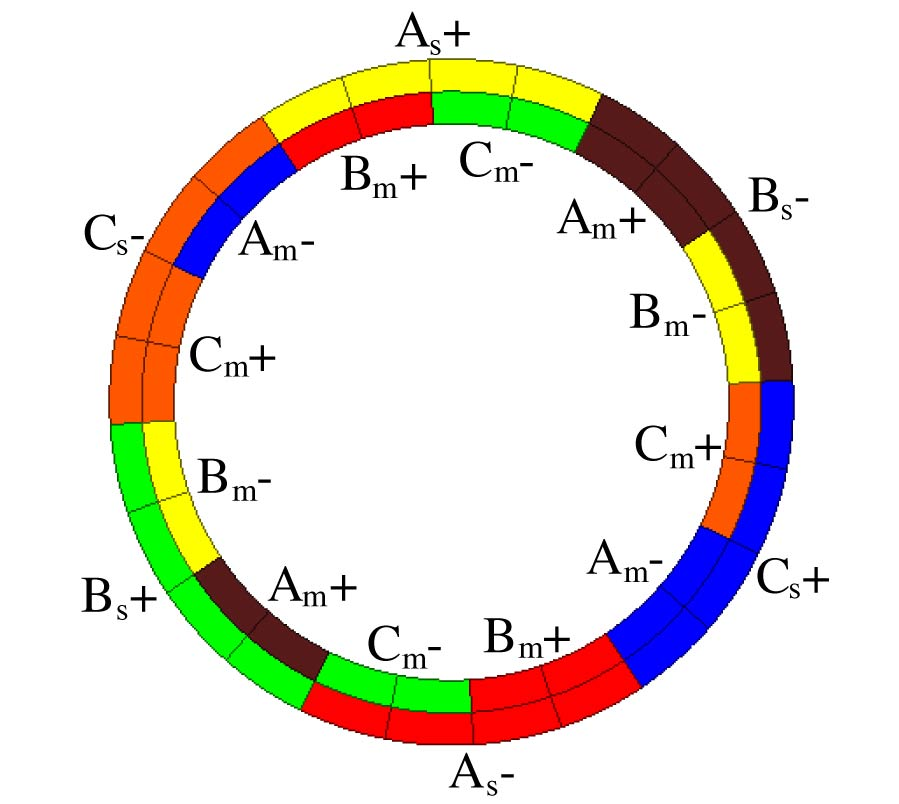2016-10-30
PIER M
Vol. 51, 139-146, 2016
Efficient Localization Algorithm of Mixed Far-Field and Near-Field Sources Using Uniform Circular Array
Bing Xue , Guangyou Fang and Yi-Cai Ji
An efficient algorithm based on high-order cumulant is addressed for the scenarios where both far-field and near-field narrow-band signals may exist synchronously. The first matrix built by four-order cumulant is utilized to estimate the two dimensional direction-of-arrivals (DOAs) using the orthogonal projection matrix of the signal subspace and the virtual steering matrix. Then, the second matrix built by four-order cumulant is decomposed to get the noise subspace using the eigen decomposition. Meanwhile, a virtual steering matrix is used to distinguish far-field signals (FFSs) from near-field signals (NFSs). And one-dimensional MUSIC algorithm is used to estimate the range of the NFSs. Compared to the TSMUSIC, the proposed algorithm can provide high resolution for the DOAs. In addition, there is higher accuracy for the DOA of NFS in the proposed algorithm than that in TSMUSIC and in TSMD. Simulation results are carried out to certify the performance of the proposed algorithm.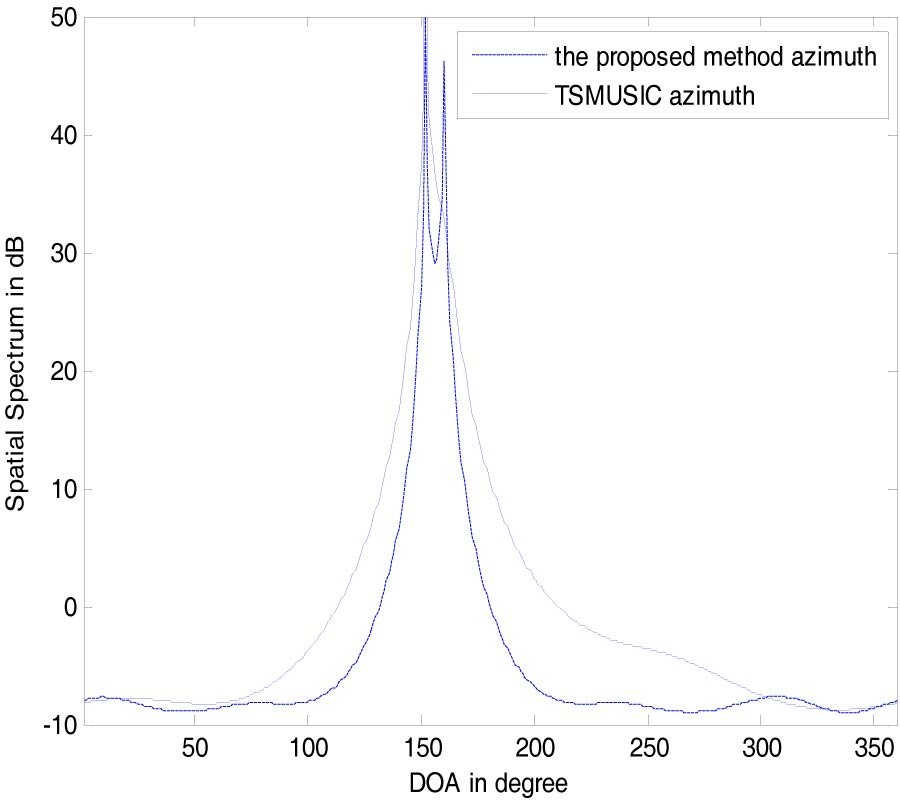2016-10-30
PIER M
Vol. 51, 131-138, 2016
Giant Faraday Rotation in One-Dimensional Photonic Crystal with Magnetic Defect
Svetlana V. Eliseeva , Yuliya F. Nasedkina and Dmitrij Igorevich Sementsov
The effect of a substantial increase of the Faraday rotation angle has been investigated in a symmetrical resonator structure, which is represented by a one-dimensional photonic crystal with dielectric Bragg mirrors and a magnetically active layer placed between mirrors. In the numerical analysis, the parameters of a pure yttrium iron garnet at two wavelengths - 1.15 μm and 1.3 μm have been used. The increase in the Faraday rotation angle is caused by not only an increase of the magnetic layer thickness, but also a symmetrical increase in the number of Bragg mirrors periods.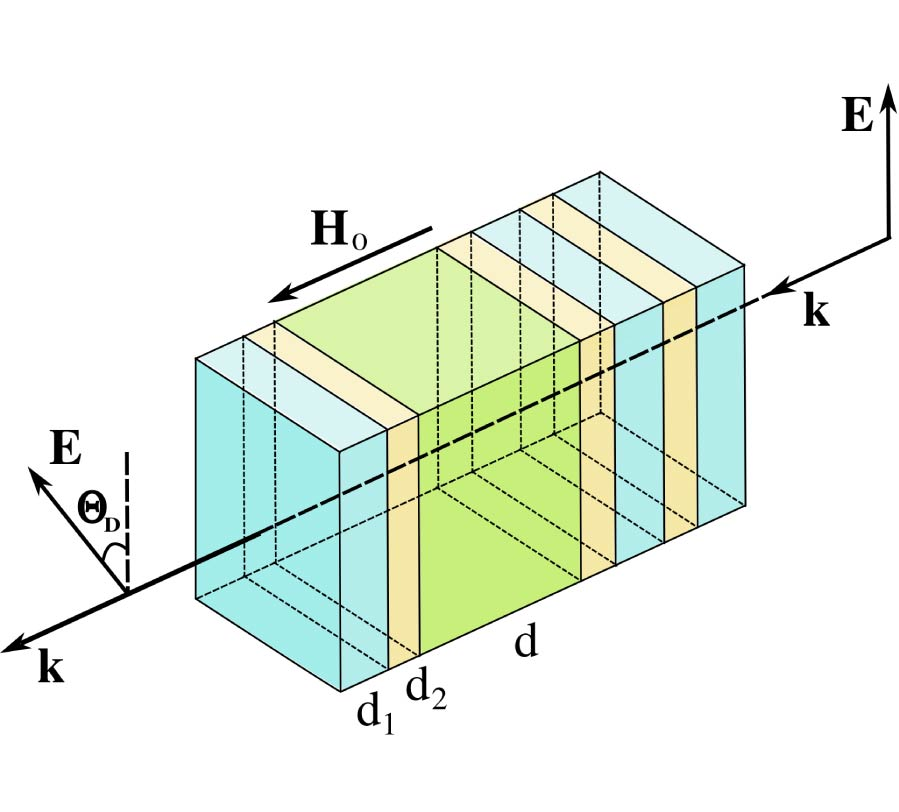2016-10-30
PIER M
Vol. 51, 121-129, 2016
Efficient Analysis of Rectangular-Shape Metamaterials Using P-CBFM/P-FFT Method
Ke Xiao , Huiying Qi , Sheng Shui Wang , Ying Liu , Liang Ding and Shun-Lian Chai
In this paper, we introduce an efficient algorithm to analyze metamaterials, which can be finite periodic structures with tightly coupling between nearby cells. Firstly, the algorithm, based on method of moments (MoM), uses hybrid volume-surface integral equation (VSIE) to analyze composite dielectric-conductor objects. Then, the characteristic basis function method (CBFM) and precorrected-fast Fourier transform (p-FFT) algorithm are combined to accelerate the calculation of equations, based on which, metamaterials composed of connected periodic cells can be analyzed efficiently.2016-10-30
PIER M
Vol. 51, 113-120, 2016
Multipactor Breakdown in Elliptical Waveguide Carrying Orthogonal Polarizations
Samaneh Esfandiarpour and Ali Frtoanpour
Multipactor effect is studied in a hollow elliptical waveguide carrying two orthogonal polarization modes, i.e., the fundamental (TEc11) and the second (TEs11) elliptical waveguide modes. The introduction of a modal equivalent voltage allows defining the standard axial ratio, which characterizes each polarization state of the problem. The RF breakdown threshold is determined as a function of the axial ratio for various amplitudes and phases of the two elliptical modes. In particular, the effect of the second mode on the RF breakdown threshold of the fundamental mode is studied. The simulations are carried out for different values of the eccentricity of the ellipse eccentricity.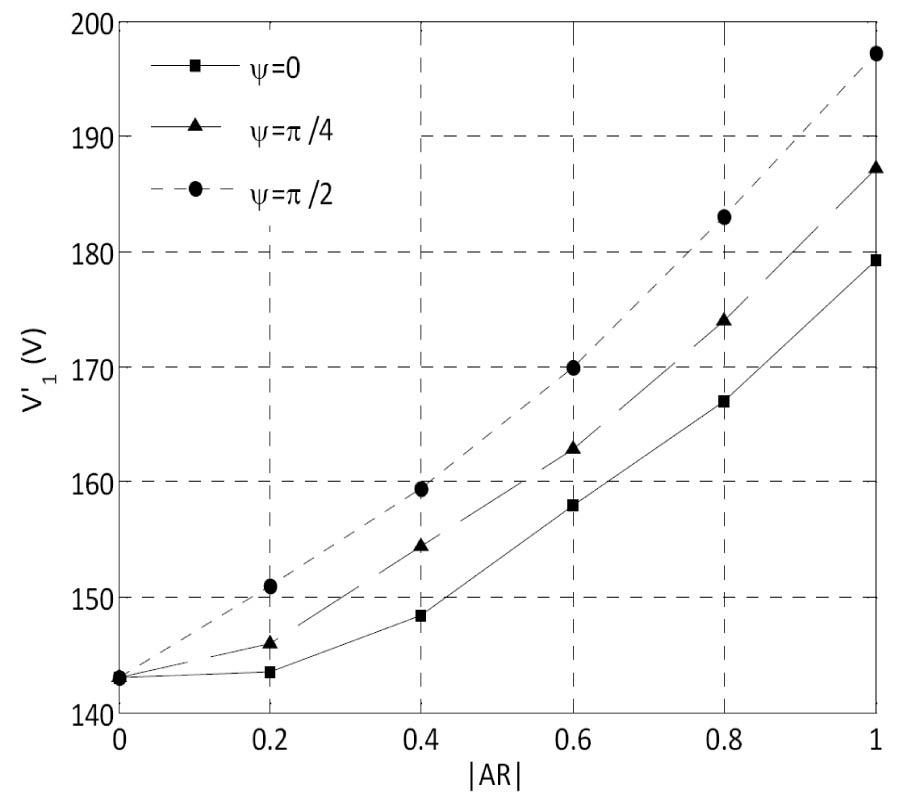2016-10-28
PIER M
Vol. 51, 101-111, 2016
Assessment of Materials for High-Speed PMSMs Having a Tooth-Coil Topology
Nikita Uzhegov , Nikolai Efimov-Soini and Juha Pyrhonen
In this paper, materials frequently used in high-speed (HS) electrical machines are assessed. Highspeed permanent magnet synchronous machines with a special tooth-coil topology serve as an example for the assessment. The lamination and rotor sleeve materials are compared taking into account their price, per unit losses, resistivity, and other factors. The resulting tables provide the electrical machine designer with a means to enhance the HS machine performance at low costs.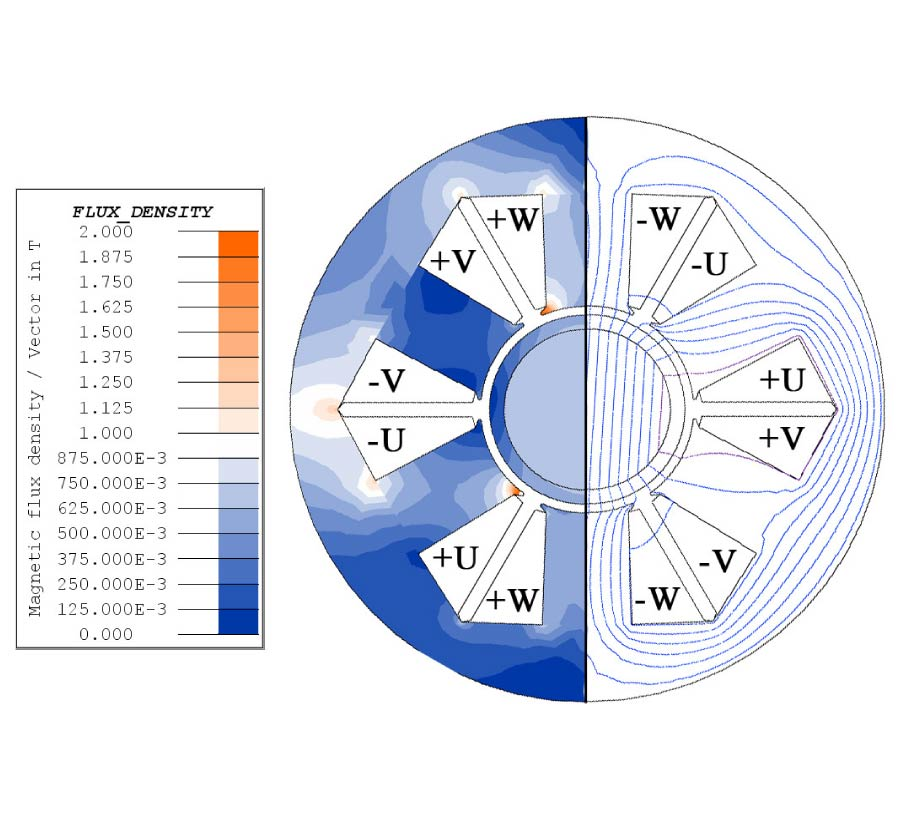2016-10-24
PIER M
Vol. 51, 93-100, 2016
Simple Method to Calculate the Force Between Thin Walled Solenoids
Jesus Jose Perez-Loya and Claes Urban Lundin
We have developed a simple method to calculate the axial force between concentric thin walled solenoids. To achieve this, we mapped the force between them as a function of their geometrical relations based on separation to diameter ratios. This resulted in an equation and a set of data. We used them together to calculate the axial forces between two coaxial thin walled solenoids. With this method, direct evaluation of elliptical integrals was circumvented, and the forces were obtained with a simple expression. The results were validated against existing semi-analytical solutions and measurements of force between high coercivity permanent magnets.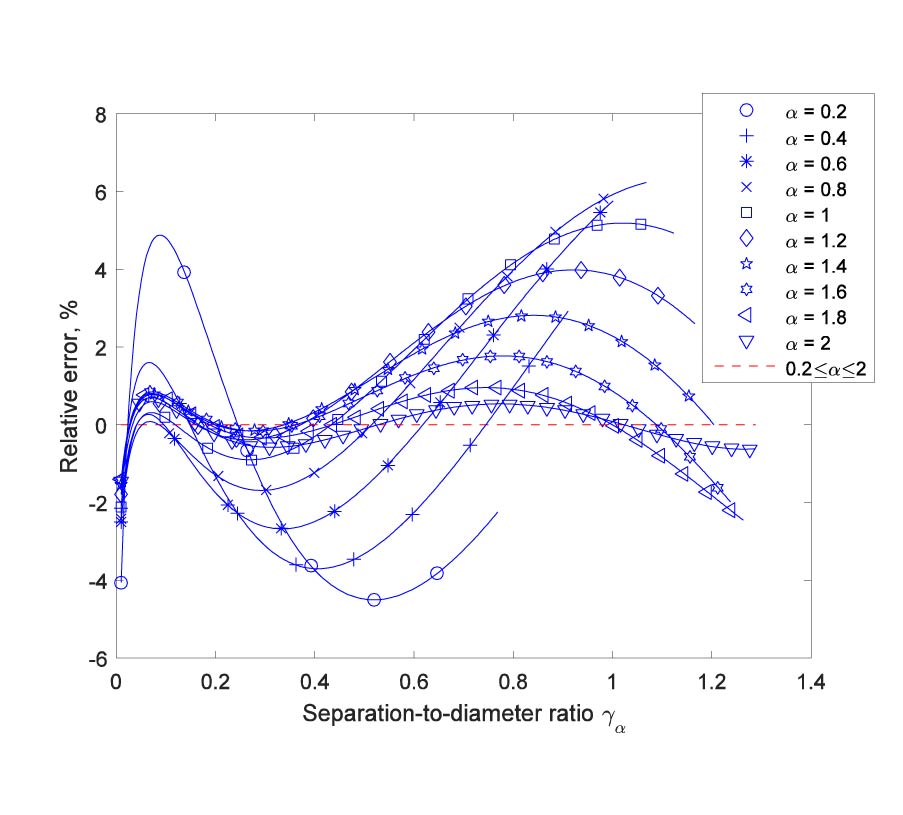2016-10-24
PIER M
Vol. 51, 83-91, 2016
An Axisymmetric Cylindrical Resonating Cavity with Hole
The problem of the shift and broadening of the normal modes of electromagnetic oscillations in a cylindrical cavity resonator with axisymmetric interior and ideally conducting walls with a circular hole at the base is solved. It is shown that the existence of the hole perturbs the normal frequencies, and this perturbation is calculated. The method of solution is based on the Rayleigh-Schrodinger perturbation theory. It is found that the frequency shift depends on the value of the perturbed electric field at the hole. This field is calculated using the quasistatic approximation, which involves the solution of a mixed boundary value problem for the potential. An expression for the frequency shift and broadening is obtained.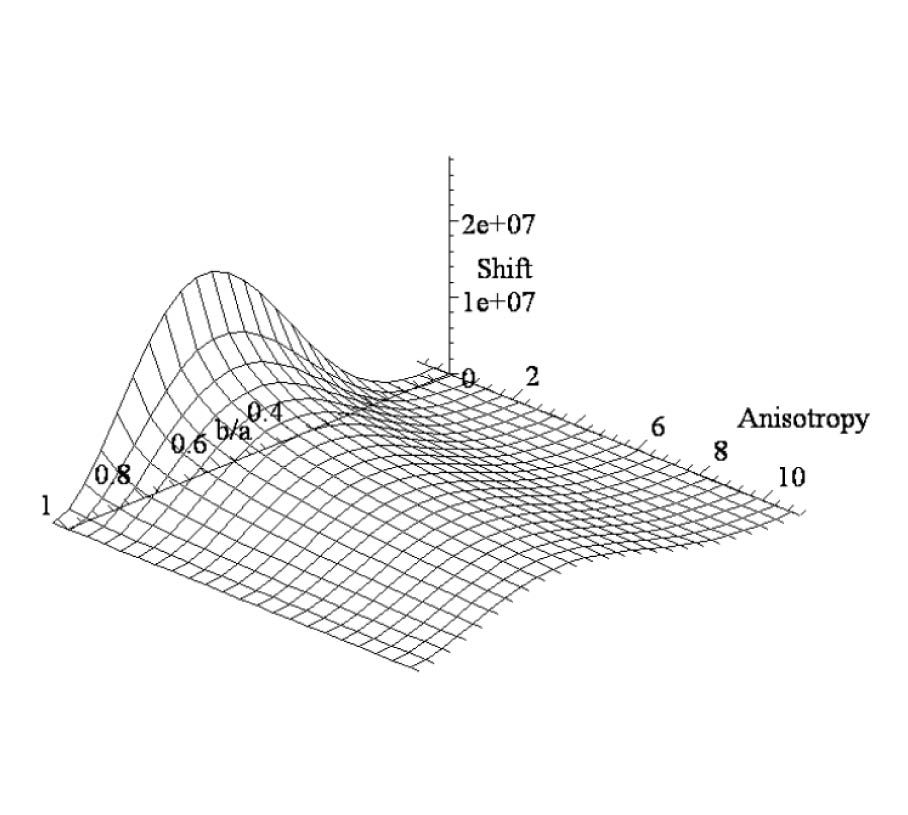2016-10-20
PIER M
Vol. 51, 71-81, 2016
Modeling of a Ku-Band Rectangular Ferrite-Loaded Waveguide Based on Left-Handed Metamaterial
Junfeng Yao , Fan Yang , Chunli Li , Degang Gan , Bing Gao and Ammad Jadoon
This paper presents the modeling and simulation of a new rectangular ferrite-loaded waveguide based on left-handed metamaterial (LHM) unit cells at Ku-band. The structure has an 8×8 unit cell configuration, whose negative permittivity and negative permeability are achieved by metallic wires array and ferrite medium, respectively. The equivalent circuit model and transmission parameter matrix for the unit cell are presented based on microwave two-port network theory. The operating frequency is in the TE10 single mode range at 12.97-15.90 GHz where magnetic and electric resonances are coupled simultaneously. The finite-element method (FEM) based simulation software HFSS has been used to set original model and optimized model with vacuum layers for decoupling. Analysis of 3D electromagnetic waves propagation and scattering parameters demonstrate the backward wave property of the optimized waveguide. Negative propagation constant and negative index of refraction are calculated based ona method for extracting effective parameters of LHM. The proposed structure has scalability, double negative, and broad-band operation characteristics in the electromagnetic paradigm.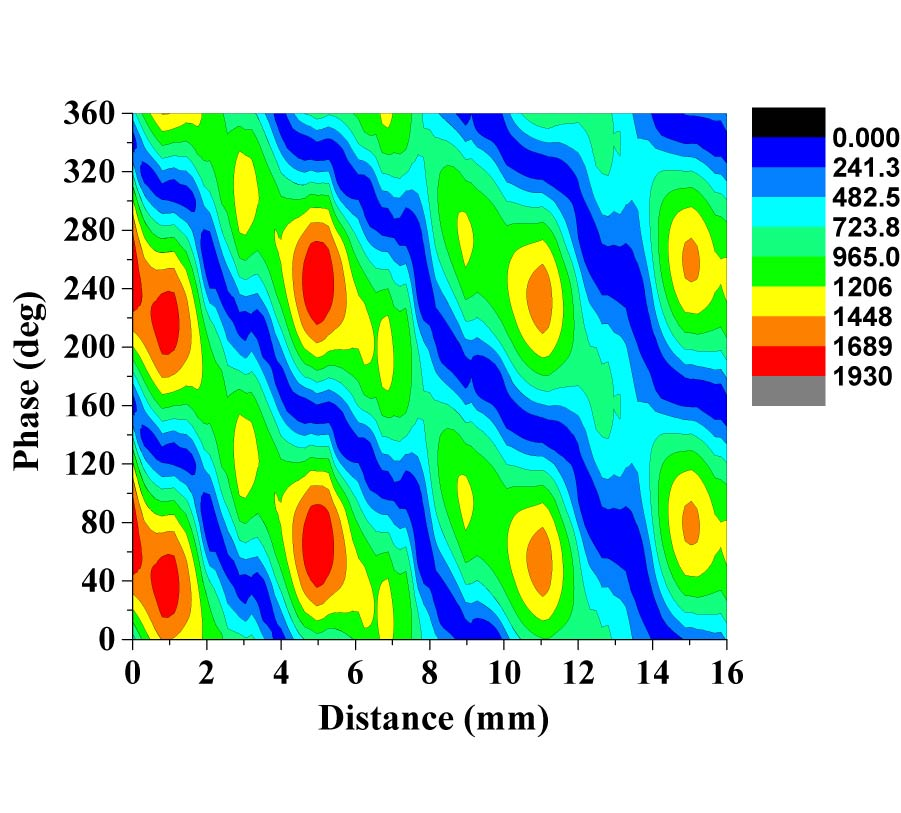2016-10-19
PIER M
Vol. 51, 63-70, 2016
Numerical Constructions of Testing Functions for Improving the Accuracy of MFIE and CFIE in Multi-Frequency Applications
Bariscan Karaosmanoglu , Aşkın Altınoklu and Ozgur Ergul
We present a new approach based on numerical constructions of testing functions for improving the accuracy of the magnetic-field integral equation (MFIE) and the combined-field integral equation (CFIE) with low-order discretizations. Considering numerical solutions, testing functions are designed by enforcing the compatibility of the MFIE systems with the accurate coefficients obtained by solving the electric-field integral equation (EFIE). We demonstrate the accuracy improvements on scattering problems, where the testing functions are designed at a single frequency and used in frequency ranges to benefit from the design procedure. The proposed approach is easy to implement by using existing codes, while it improves the accuracy of MFIE and CFIE without deteriorating the efficiency of iterative solutions.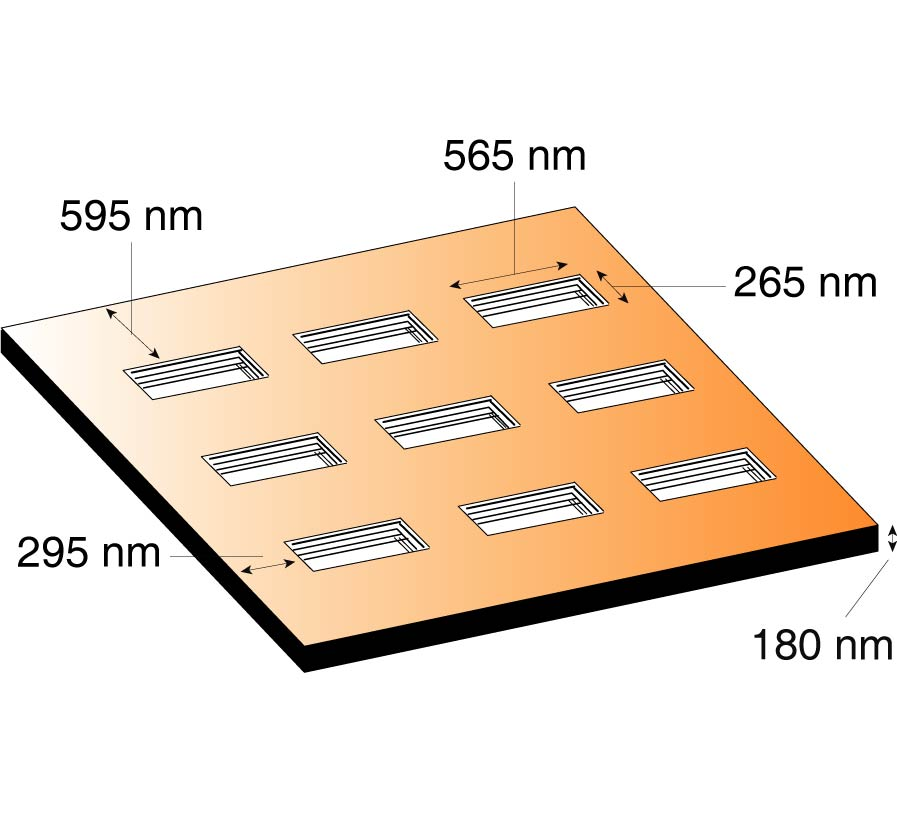2016-10-17
PIER M
Vol. 51, 51-62, 2016
Simulation of Multi-Layer Rough Surfaces Media in the Passive Millimeter-Wave Imaging
Chuan Yin , Ming Zhang and Yaming Bo
The simulation of multi-layer rough surfaces is an indispensable step in passive radiation imaging, to which little attention has been paid so far. Based on the existing model of brightness temperature tracing described in our recent works, diffused transmission of the bottom layer is taken into account in the improved model which is presented in this paper. Then, a method called multi-layer brightness temperature tracing method (MBTT) is established to obtain the brightness temperature of a rough surface, and the applied range of the simulation in passive millimeter-wave imaging (PMMW) is extended.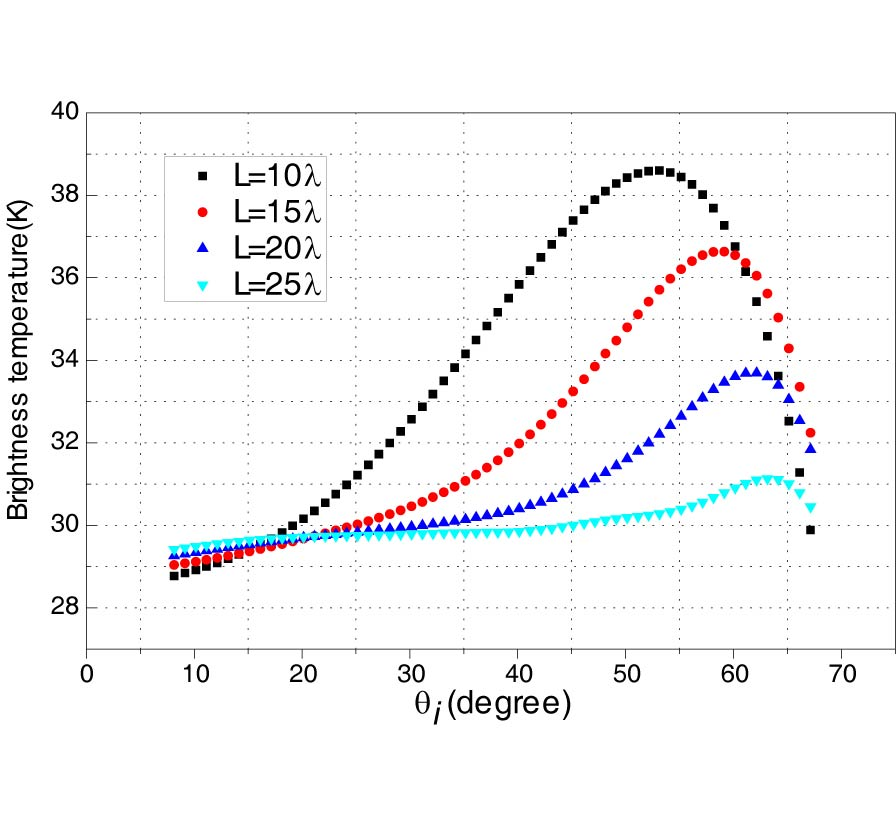2016-10-14
PIER M
Vol. 51, 33-50, 2016
Characterization of Linear Electromagnetic Observables in Stochastic Field-to-Wire Couplings
Ousmane Oumar Sy , Martijn Constant van Beurden and Bastiaan L. Michielsen
This article presents a method to characterize stochastic observables defined by induced surface currents and fields in electromagnetic interactions with uncertain configurations. As the covariance operators of the stochastic distributions and fields are not compact, a strict Karhunen-Loeve (KL) approach is not possible. Instead, we apply a point-spectrum regularization by expanding the stochastic quantities on a finite-element-like basis. The coefficients of the KL expansion are approximated analytically in a polynomial-chaos (PC) expansion. The novelty of our approach resides in its ability to handle multiple PC expansions simultaneously and determine the orders of the KL and PC expansions adaptively. Thismethod is illustrated through the example of the voltage induced at the port of a random thin-wire frame illuminated by random plane waves. The results show the accuracy and computational efficiency of the proposed method, which provides a complete characterization of the randomness of the observable.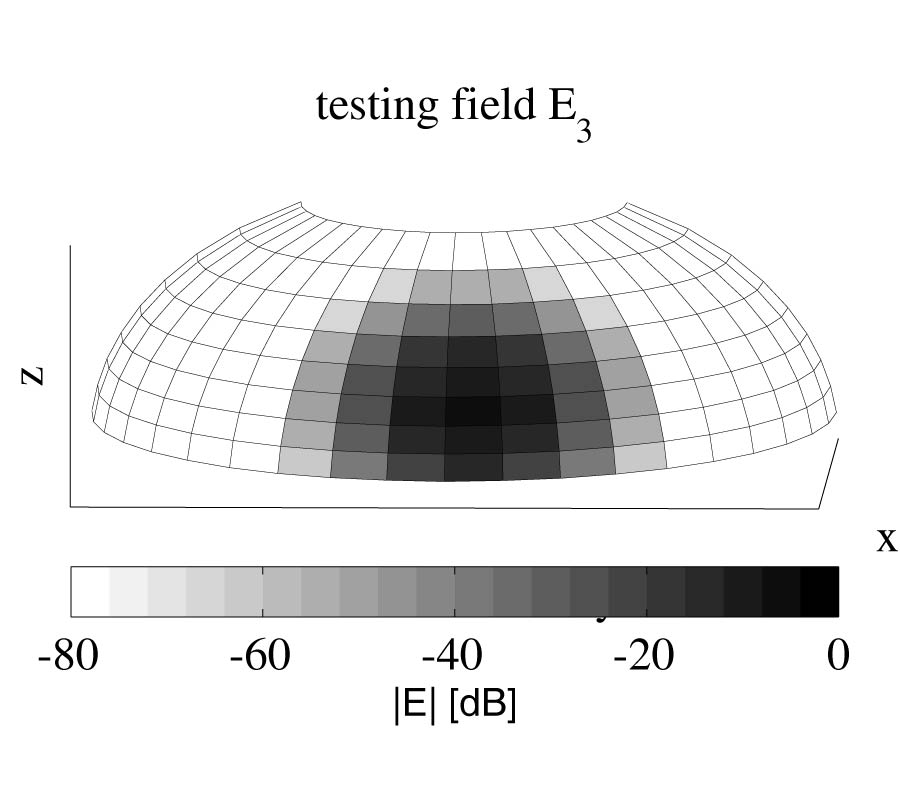2016-10-14
PIER M
Vol. 51, 19-31, 2016
Retrieval of Refractivity Profile with Ground-Based Radio Occultation by Using an Improved Harmony Search Algorithm
Mu-Min Chiou and Jean-Fu Kiang
A ground-based radio occultation (RO) technique is proposed to retrieve the atmospheric refractivity profile around a specific region at a higher sampling rate than conventional space-based RO techniques, making it more suitable for regional weather studies. A harmony search (HS) algorithm with ensemble consideration (HS-EC) based on atmospheric physics is proposed to retrieve the refractivity profile more efficiently without being trapped in suboptimal solutions. The highest altitude of profile is extended to 95 km from 40 km adopted in conventional ground-based RO techniques, leading to more accurate results.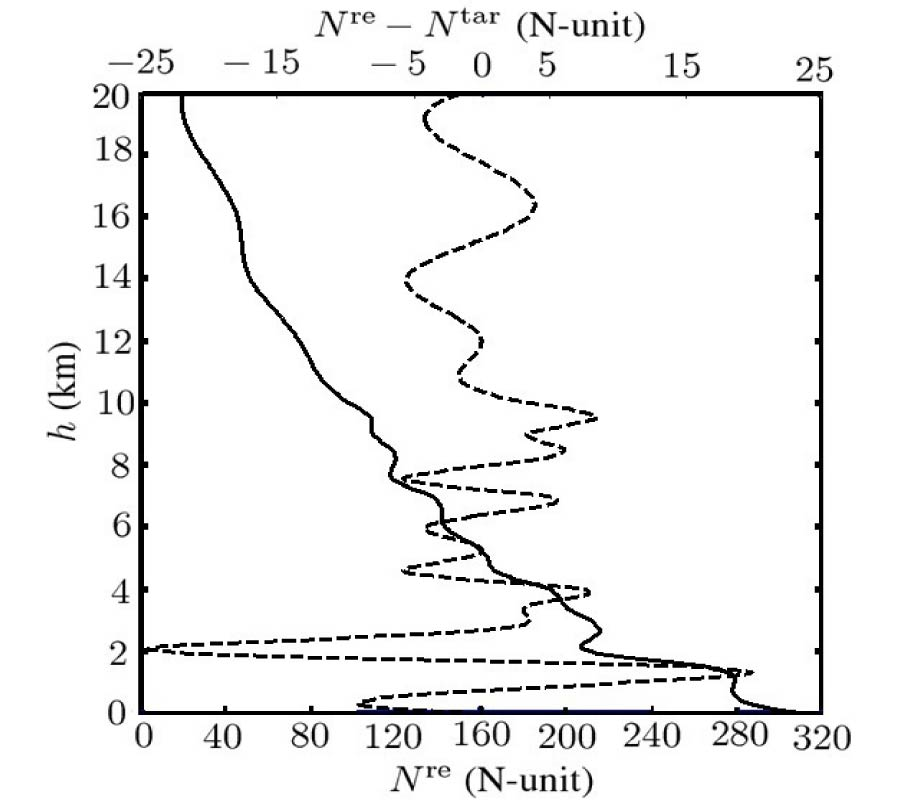2016-10-13
PIER M
Vol. 51, 9-17, 2016